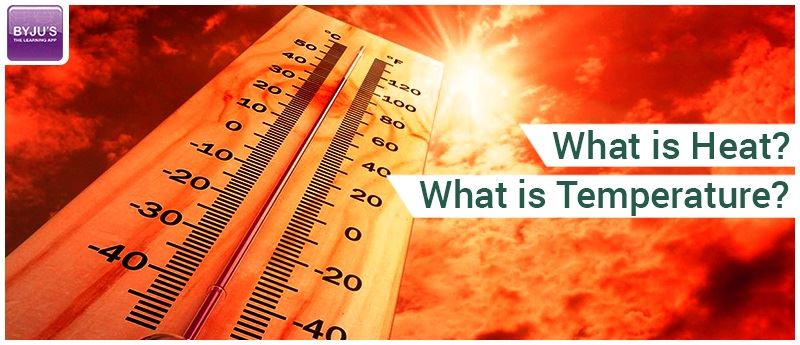# Difference Between Heat and Temperature

We often talk about heat and temperature in our daily life in terms of weather or sometimes in terms of temperature. But do we know the difference between heat and temperature? It is important to realize the minute difference between heat and temperature scales for measuring the hotness or coldness of any object.

## Heat vs Temperature

Heat and temperature are interconnected, but contrary to popular belief, they do not mean the same thing. Knowing the distinction between heat and temperature can lead to a clearer understanding of the world around us. In this article, we will define both heat and temperature and reach an understanding of how they are related, but not identical.$$\begin{array}{l}Heat ≠ Temperature\end{array}$$

The reason why the concept of heat and temperature might be mixed up is because of how closely they are related in real life. If you add heat to something, its temperature goes up. If you reduce temperature, you are taking its heat away. Let’s look at what they actually represent.

Heat is the total energy of the motion of the molecules of a substance, whereas temperature refers to the measure of the average energy of the motions of the molecules in the substance. The heat is dependent on factors like the speed of the particles, the size of the particles and the number of particles, etc.

The temperature, on the other hand, is independent of these factors. For example, let’s compare a tub of water with a cup of water. The water in the tub and the cup can be at the same temperature but by the virtue of the number of particles in the tub, the water in the tub has more thermal energy in it and thereby more heat even though they have the same temperature.

Temperature is a number that relates to the energy possessed by the molecules of a substance, which directly relates to the kinetic energy possessed by the particles of the substance. The SI Unit of temperature is Kelvin. As we said earlier, the temperature is directly related to the kinetic energy of the molecules, therefore if you double the temperature (Kelvin) of a substance, you double the average kinetic energy possessed by those molecules.

### Comparison Chart

 Quantity Used to Compare Heat Temperature Definition The heat of an object is the total energy of all the molecular motion inside that object. Temperature is the measure of the thermal energy or average heat of the molecules in a substance. SI Unit Joule Kelvin Unit Joules, Calories Fahrenheit, Celsius, Kelvin Symbol Q T Particles It’s a measure of the number of atoms multiplied by the energy possessed by each atom. It is like a level which determines the direction in which the heat will flow. It is related to how fast the molecules within a substance are moving. Ability to do Work It has the ability to do work It can be used to measure the degree of heat.

Let’s take a closer look at heat and temperature now. When you add heat to a substance you are giving it energy. There are two things that can happen when you heat a substance;

• The substance undergoes a rise in temperature corresponding to the increase in the kinetic energy of the molecules; i.e. the heat gives the molecules extra energy to move around with more speed, and this increase in speed implies an increase in temperature. The molecules now travel faster than before the heat was supplied.
• The substance can also undergo a change in phase or state; for example, at a certain temperature i.e. 100oC or 373oK, with the addition of further heat, the water molecules begin to leave in a vapour state. This is called the change of phase. One thing we should remember is that the energy added to change the state of a substance does not result in an increase in temperature, i.e, if we have a cup of water at 90o C, the addition of heat will cause this temperature to rise to 100o C, if we still add heat, the water turns into water vapour with a temperature of 100o The absorbed energy in phase changes is not used to increase the temperature but is used to change the phase and it is called the latent heat of vaporization.## Frequently Asked Questions – FAQs

### What is heat?

Heat is the energy that is transferred from one body to another due to differences in temperature.

### What is temperature?

Temperature is a physical quantity that expresses the coldness and hotness of a body.

### What is the SI unit of temperature?

The SI unit of temperature is Kelvin.

True.

### What is the SI unit of heat?

The SI unit of heat is Joule.

Stay tuned with BYJU’S to learn more about the Difference Between Heat and Temperature, heat energy and much more.

Test your knowledge on Heat and temperature differences